Courses

# Sampling, JIT, TQM And Graphical Method - MCQ Test 1

## 15 Questions MCQ Test Mock Test Series - Mechanical Engineering (ME) for GATE 2020 | Sampling, JIT, TQM And Graphical Method - MCQ Test 1

Description
This mock test of Sampling, JIT, TQM And Graphical Method - MCQ Test 1 for Mechanical Engineering helps you for every Mechanical Engineering entrance exam. This contains 15 Multiple Choice Questions for Mechanical Engineering Sampling, JIT, TQM And Graphical Method - MCQ Test 1 (mcq) to study with solutions a complete question bank. The solved questions answers in this Sampling, JIT, TQM And Graphical Method - MCQ Test 1 quiz give you a good mix of easy questions and tough questions. Mechanical Engineering students definitely take this Sampling, JIT, TQM And Graphical Method - MCQ Test 1 exercise for a better result in the exam. You can find other Sampling, JIT, TQM And Graphical Method - MCQ Test 1 extra questions, long questions & short questions for Mechanical Engineering on EduRev as well by searching above.
QUESTION: 1

Solution:
QUESTION: 2

Solution:
QUESTION: 3

### Preliminary work sampling studies show that machine was idle 25% of the time based on a sample of 100 observations. The number of observations needed for a confidence level of 95% and an accuracy of ± 5% is:

Solution: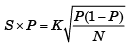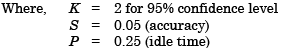Number of observations needed for 95% confidence level and ± 5% accuracy is :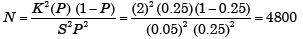QUESTION: 4

If at the optimum in a linear programming problem, a dual variable corresponding to a particular primal constraint is zero, then it means that

Solution:
QUESTION: 5

Which one of the following correctly explains process capability?

Solution:
QUESTION: 6

Consider the following Linear Programming Problem (LPP):
Maximize z = 3x1 + 2x2
Subject to x1 ≤ 4
x2 ≤ 6
3x1 + x2 ≤ 18
x2 ≥ 0, x2 ≥ 0

Solution:
QUESTION: 7

Process capability of a machine is defined as the capability of the machine to:

Solution:

Process capability of a machine is defined as the capability of the machine to hold a definite spectrum of tolerances and surface finish.

QUESTION: 8

A manufacturer produces two types of products, 1 and 2, at production levels of x1 and x2 respectively. The profit is given is 2x1 + 5x2. The production constraints are: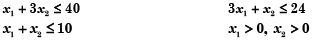The maximum profit which can meet the constraints is:

Solution:

Rearranging the above equations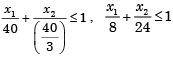and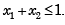Draw the lines and get solution.

QUESTION: 9

Match List-I with List-II and select the connect answer using the codes given below the lists: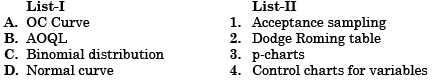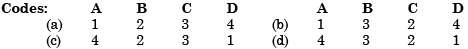Solution:
QUESTION: 10

Which one of the following is the correct statement?

In the standard form of a linear programming problem, all constraints are:

Solution:
QUESTION: 11

The curve representing the level of achievement with reference to time is known as

Solution:
QUESTION: 12

Match List-I with List-II and select the correct answer using the codes given below the lists: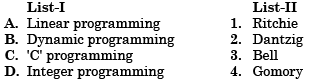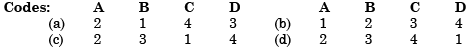Solution:
QUESTION: 13

An operating characteristic curve (OC curve) is a plot between

Solution:

OC Curve (Operating Characteristic Curve)
OC CURVE is a plot between percentage of defective and probability of acceptance.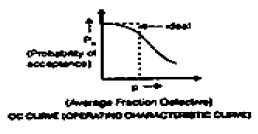QUESTION: 14

A feasible solution to the linear programming problem should

Solution:

A feasible solution to the linear programming problem should satisfy the problem constraints.

QUESTION: 15

Assertion (A): Double sampling is preferred over single sampling when the quality or incoming lots is expected to be either very good or very bad.

Reason (R): With double sampling, the amount of inspection required will be lesser than that in the case of single sampling.

Solution: